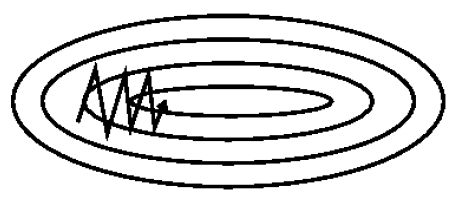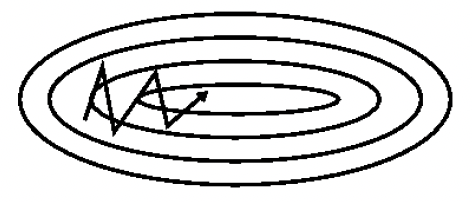# Momentum¶

$v_{t}=\gamma v_{t-1}+\eta \nabla J(\theta)$

SGD-M参数更新公式如下，其中$$\eta$$是学习率，$$\nabla J(\theta)$$是当前参数的梯度

$\theta=\theta-v_{t}$momentum能够加速SGD方法，并且能够减少震荡，如下图：• 加入了动量因素，SGD-M缓解了SGD在局部最优点梯度为0，无法持续更新的问题和振荡幅度过大的问题。

• 当局部沟壑比较深，动量加持用完了，依然会困在局部最优里来回振荡